Function Repository Resource:

# TimeShiftedDistribution

A derived distribution useful in actuarial science

Contributed by: Seth J. Chandler
 ResourceFunction["TimeShiftedDistribution"][xmin,dist] represents a distribution dist that has been truncated to lie above xmin and then translated so that the lowest outcome is 0. ResourceFunction["TimeShiftedDistribution"][{xmin,ymin,…},dist] represents a multivariate truncation of the distribution dist, translated so the lowest outcome from the distribution is a vector of 0s.

## Details and Options

The translation works by subtracting xmin from the outputs so that the lowest outcome from the distribution is now 0.
A time-shifted distribution can be used to model the longevity of a person who had a certain longevity distribution at birth but who has survived to some attained age.

## Examples

### Basic Examples (2)

A BinomialDistribution, time-shifted so that its value must lie above 3 and be translated to the left by 3:

 In:=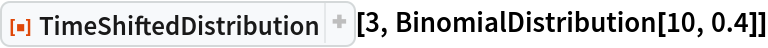Out=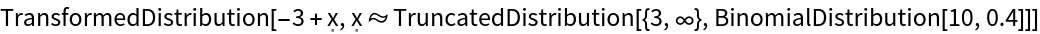The probability density (mass) function of that distribution:

 In:=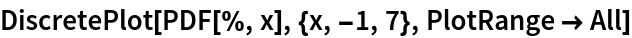Out=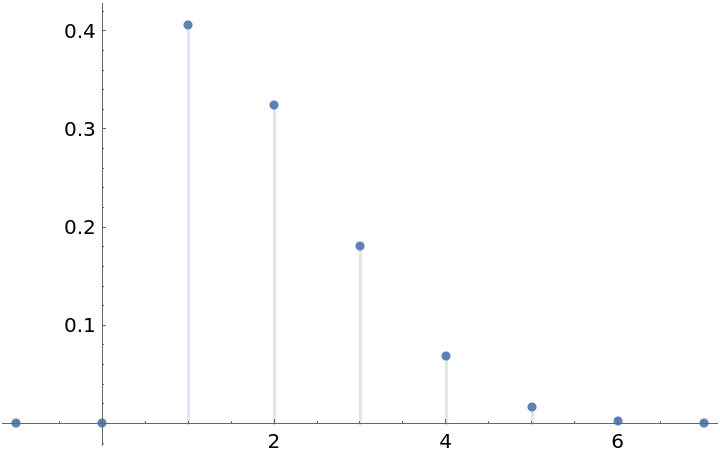The distribution of the remaining life of a person aged 61, where longevity at birth is distributed according to a GompertzMakehamDistribution:

 In:=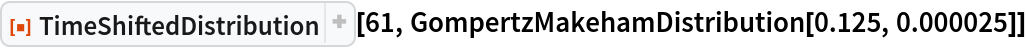Out=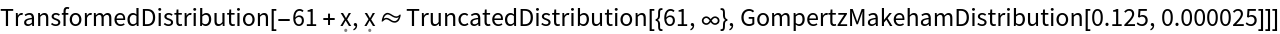The survival function of that time-shifted distribution:

 In:=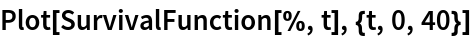Out=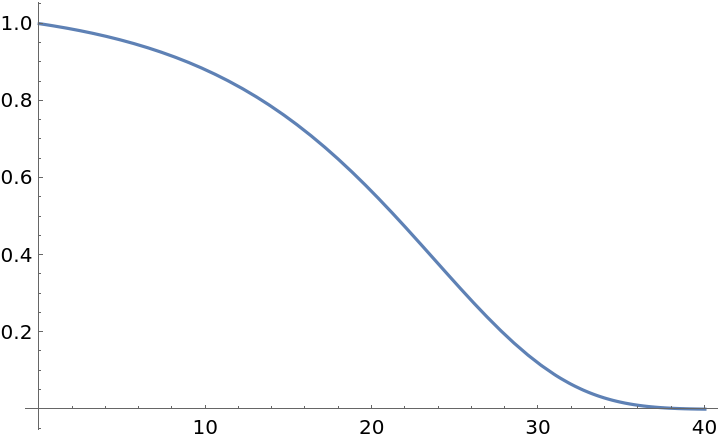### Scope (2)

The mean of a time-shifted distribution of a ProductDistribution of two symbolic BetaDistributions:

 In:=Out=A random variable drawn from a time-shifted MultinormalDistribution:

 In:=Out=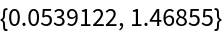The numeric probability of a draw from a time-shifted CopulaDistribution falling into a particular set of values:

 In:=Out=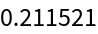### Applications (2)

Show survival functions of a BetaDistribution for different time-shift values:

 In:=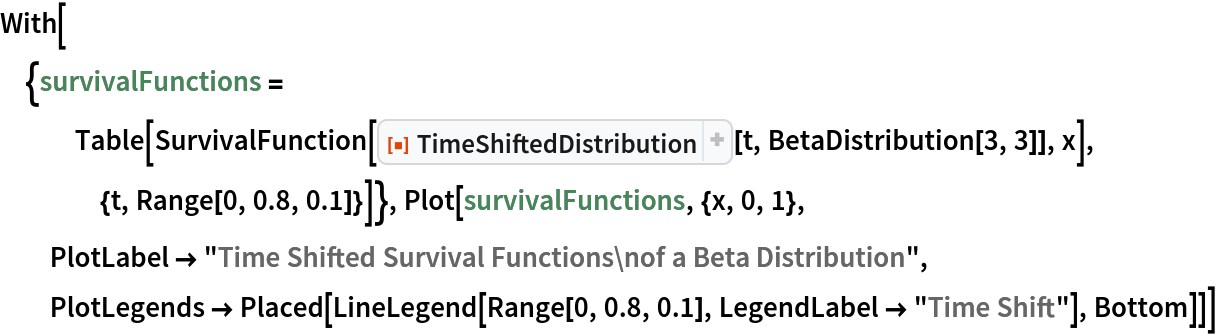Out=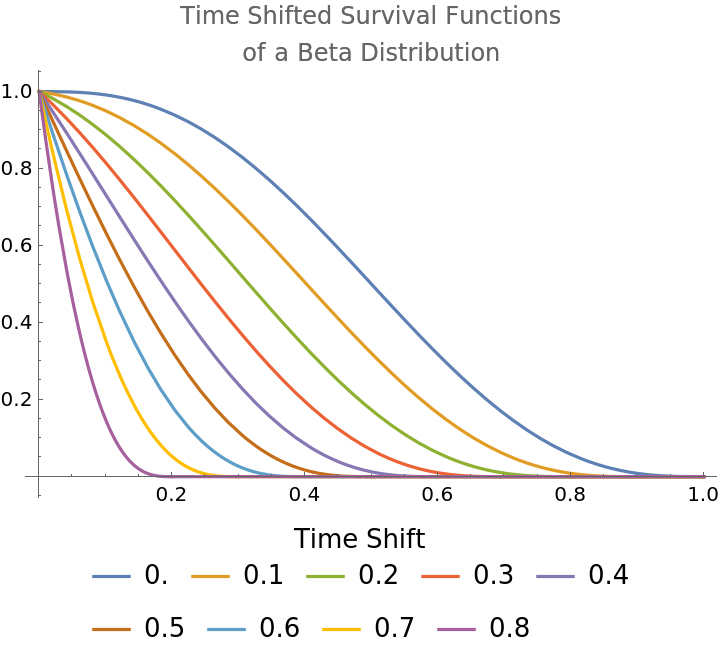Show survival functions of a GompertzMakehamDistribution for different time-shift values:

 In:=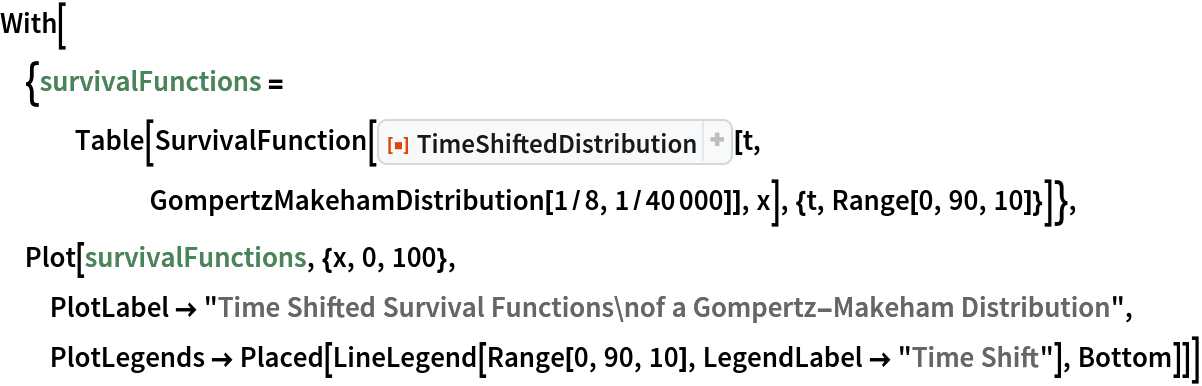Out=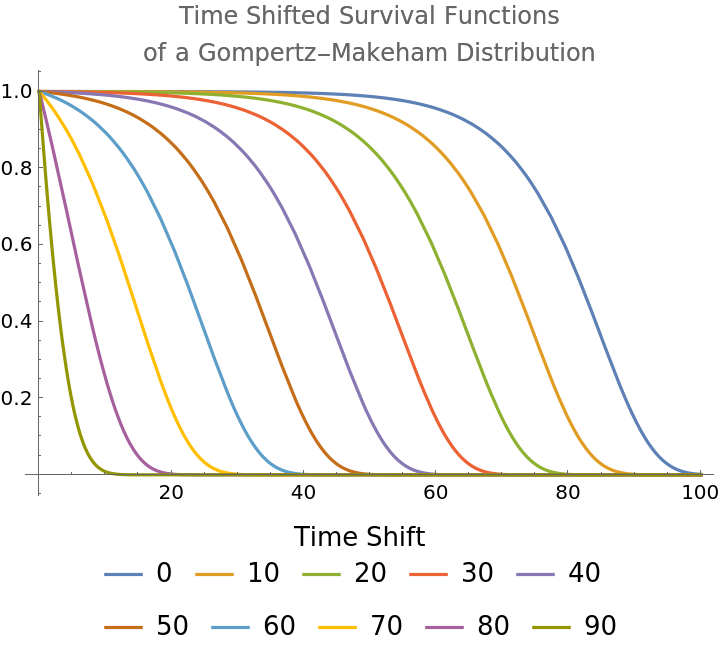### Properties and Relations (1)

Some distributions, such as the ExponentialDistribution, remain unchanged after time shifting :

 In:=Out=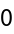### Neat Examples (3)

Consider a life insurance product in which the time a person dies and the time a person lets their policy lapse are given by the ProductDistribution of a GompertzMakehamDistribution (for longevity) and an ExponentialDistribution (for lapse). The insured has neither died nor lapsed before age 61. Compute the probability that an insurer will have to pay a death benefit between k and k+1 years thereafter and that the insurance policy will not have lapsed by that time:

 In:=In:=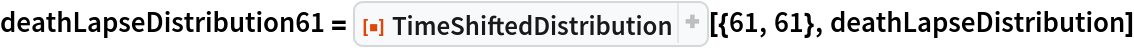Out=In:=Out=Compute the actuarial present value of a death benefit of \$1 for such a person, assuming the policy pays nothing if the policy lapses before death and assuming a 5% effective rate of interest:

 In:=Out=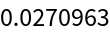Compute the maximum amount the insurer would be willing to pay a 75-year-old who holds such a policy to let it lapse, again assuming a 5% effective rate of interest:

 In:=Out=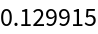Seth J. Chandler

## Version History

• 1.0.0 – 07 August 2019